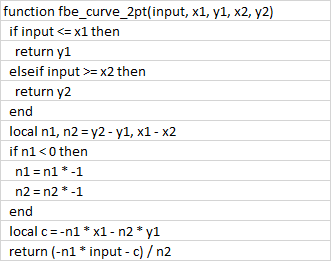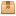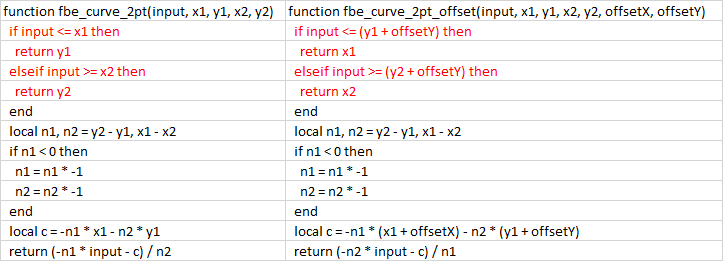FB EditorKoBraJunior Member Posts: 38 Threads: 7 Joined: Apr 2018 Reputation: 1 12.01.2021, 10:38 I found in the latest release 20201210 FB Editor 2.0 the function to calculate a sliding setpoint with or without offset. I tried it but there seems a bug in the one with offset:Does somebody also know why the settings are only available as value and not object? For installers i normally make the X and Y value programmable. For end user normally only the Offset on Y is a setting (but this is also only available as value).Daniel.Senior Member Posts: 2351 Threads: 15 Joined: Aug 2017 Reputation: 90 12.01.2021, 10:50 This is defined in the function which defines this block. Here are all the function https://forum.logicmachine.net/showthrea...49#pid4849. You can import them to user libraries and modify. This is quite old one and not sure if this is same like in the current FB.KoBraJunior Member Posts: 38 Threads: 7 Joined: Apr 2018 Reputation: 1 12.01.2021, 12:20 (This post was last modified: 12.01.2021, 12:22 by KoBra.) Nope i checked and tested this one has the bug in both. So it seems the curve with 2 points function is updated sometime ago. with the data above the outcome for both is now -10Daniel.Senior Member Posts: 2351 Threads: 15 Joined: Aug 2017 Reputation: 90 12.01.2021, 12:23 I will try to get updated libs but it was done buy external dev so it might take some time.KoBraJunior Member Posts: 38 Threads: 7 Joined: Apr 2018 Reputation: 1 13.01.2021, 09:15 I reverse engineered it and used some excel to test the calculations and the one below works. --- [Function] fbe_curve_2pt --- Curve function with 2 points --- [Comment] --- Depending on a straight line defined by 2 points, this module supplies a y-value for an x-value. --- The first point of the straight line is defined by x1 and y1. --- The second point of the straight line is defined by x2 and y2. --- If x is less than x1, then y=y1. --- If x is greater than x2, then y=y2. --- Only strictly monotone rising or falling functions can be realised. This means x1 < x2. --- [Input] --- Input [value, object, storage] --- X1 [value, object, storage] --- Y1 [value, object, storage] --- X2 [value, object, storage] --- Y2 [value, object, storage] --- [Output] --- out - Output [object, storage] function fbe_curve_2pt(input, x1, y1, x2, y2) if input <= x1 then return y1 elseif input >= x2 then return y2 end local n1, n2 = y2 - y1, x1 - x2 if n1 < 0 then n1 = n1 * -1 n2 = n2 * -1 end local c = n1 / n2 return y2 + (x2 - input) * c end --- [Function] fbe_curve_2pt_offset --- Curve function with 2 points and offset --- [Comment] --- Depending on straight lines defined by 2 points, this module supplies an x-value for a y-value. --- The first point of the straight line is defined by x1 and y1. --- The second point of the straight line is defined by x2 and y2. --- The x and the y-axis can be shifted by the respective offset. --- If x is less than (x1 offset x), then y=y1. --- If x is greater than (x2 offset x), then y=y2. --- [Input] --- Input [object, storage] --- X1 [value, object, storage] --- Y1 [value, object, storage] --- X2 [value, object, storage] --- Y2 [value, object, storage] --- Offset X [value, object, storage] --- Offset Y [value, object, storage] --- [Output] --- out - Output [object, storage] function fbe_curve_2pt_offset(input, x1, y1, x2, y2, offsetX, offsetY) if input <= (x1 + offsetX) then return (y1 + offsetY) elseif input >= (x2 + offsetX) then return (y2+ offsetY) end local n1, n2 = y2 - y1, x1 - x2 if n1 < 0 then n1 = n1 * -1 n2 = n2 * -1 end local c = n1 / n2 return (y2 + offsetY) + ((x2 + offsetX) - input) * c endDaniel.Senior Member Posts: 2351 Threads: 15 Joined: Aug 2017 Reputation: 90 14.01.2021, 10:46 Libraries from latest FB Attached FilesAlerts.tar.gz (Size: 1.21 KB / Downloads: 2)Comparator.tar.gz (Size: 1.93 KB / Downloads: 1)Control.tar.gz (Size: 7.88 KB / Downloads: 2)Converter.tar.gz (Size: 4.84 KB / Downloads: 1)DALI.tar.gz (Size: 921 bytes / Downloads: 1)Filter.tar.gz (Size: 743 bytes / Downloads: 1)Gate.tar.gz (Size: 1.79 KB / Downloads: 1)Math_advanced.tar.gz (Size: 4.3 KB / Downloads: 3)Math_basic.tar.gz (Size: 1.83 KB / Downloads: 1)Select_switch.tar.gz (Size: 1.66 KB / Downloads: 1)String.tar.gz (Size: 2.95 KB / Downloads: 1)Time.tar.gz (Size: 6.06 KB / Downloads: 2)KoBraJunior Member Posts: 38 Threads: 7 Joined: Apr 2018 Reputation: 1 14.01.2021, 11:44 (14.01.2021, 10:46)Daniel. Wrote: Libraries from latest FBI found the problem, without the offset the X is the input and the Y the output. In the one with offset the Y is the input and the X the output...KoBraJunior Member Posts: 38 Threads: 7 Joined: Apr 2018 Reputation: 1 14.01.2021, 12:53 The adapted script, where in both versions the X is input (outdoor air temperature for example) and the calculated Y is output. --- [Function] fbe_curve_2pt --- Curve function with 2 points --- [Comment] --- Depending on a straight line defined by 2 points, this module supplies a y-value for an x-value. --- The first point of the straight line is defined by x1 and y1. --- The second point of the straight line is defined by x2 and y2. --- If x is less than x1, then y=y1. --- If x is greater than x2, then y=y2. --- Only strictly monotone rising or falling functions can be realised. This means x1 < x2. --- [Input] --- Input [value, object, storage] --- X1 [value, object, storage] --- Y1 [value, object, storage] --- X2 [value, object, storage] --- Y2 [value, object, storage] --- [Output] --- out - Output [object, storage] function fbe_curve_2pt(input, x1, y1, x2, y2) if input <= x1 then return y1 elseif input >= x2 then return y2 end local n1, n2 = y2 - y1, x1 - x2 if n1 < 0 then n1 = n1 * -1 n2 = n2 * -1 end local c = -n1 * x1 - n2 * y1 return (-n1 * input - c) / n2 end --- [Function] fbe_curve_2pt_offset --- Curve function with 2 points and offset --- [Comment] --- Depending on straight lines defined by 2 points, this module supplies an x-value for a y-value. --- The first point of the straight line is defined by x1 and y1. --- The second point of the straight line is defined by x2 and y2. --- The x and the y-axis can be shifted by the respective offset. --- If x is less than (x1 offset x), then y=y1. --- If x is greater than (x2 offset x), then y=y2. --- [Input] --- Input [object, storage] --- X1 [value, object, storage] --- Y1 [value, object, storage] --- X2 [value, object, storage] --- Y2 [value, object, storage] --- Offset X [value, object, storage] --- Offset Y [value, object, storage] --- [Output] --- out - Output [object, storage] function fbe_curve_2pt_offset(input, x1, y1, x2, y2, offsetX, offsetY) if input <= (x1 + offsetX) then return y1 elseif input >= (x2 + offsetX) then return y2 end local n1, n2 = y2 - y1, x1 - x2 if n1 < 0 then n1 = n1 * -1 n2 = n2 * -1 end local c = -n1 * (x1 + offsetX) - n2 * (y1 + offsetY) return (-n1 * input - c) / n2 end « Next Oldest | Next Newest »